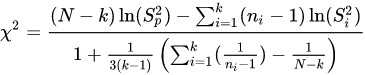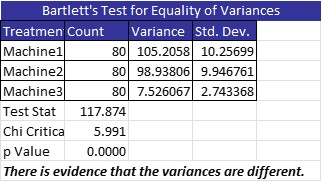The Bartlett Test is named after the English statistician, Maurice Stevenson Bartlett. The test is also known as the Bartlett’s test for homogeneity of variances. It is most used in the context of doing a 1-way Analysis of Variance (ANOVA). ANOVA is used to determine whether there is any statistical difference between the means of three or more groups of continuous data. There are two underlying assumptions for ANOVA. One is that all groups of data need to be normally distributed, and the other, is that the variances need to be statistically equal.

## Overview: What is the Bartlett Test?

You will use the Bartlett Test to determine if the variances of your data sets are equal when your data is determined to be normally distributed. A similar test, the Levene’s Test is better suited when your data is not normally distributed. The Levene test is less sensitive than the Bartlett test to departures from normality. If you have strong evidence that your data comes from a normal, or nearly normal, distribution, then Bartlett’s test has better performance.

The null hypothesis (Ho) for the Bartlett Test is that all variances are equal. The alternative hypothesis (Ha) is that at least one of your variances is different. The test statistic for the Bartlett Test is:## An industry example of the Bartlett Test

The Six Sigma Black Belt wanted to know whether the average run speed of the company’s three manufacturing machines was equal. She gathered some sample data and decided to use ANOVA to answer her question. She used a statistical software package and determined that the three sample data sets were all normally distributed. She then ran the Bartlett Test to determine whether the three variances were equal. Below is the output of her analysis:As you can see, the variances are not equal, therefore it would not be appropriate to use the standard ANOVA. Instead, you can use Welch’s ANOVA which does not require equal variances.

### What is the Bartlett Test used for?

It is used to test the hypothesis that the variances of multiple data sets are statistically equal.

### Can the Bartlett Test be used if my data sets are not normally distributed?

It can, but the Levene Test is a better choice for non-normal data sets.

### What is the null hypothesis for the Bartlett Test?

The null hypothesis is all the population variances being tested are equal. The alternative hypothesis is the population variances are not all equal. This means at least one is not equal to the others. The test does not explicitly determine which one is different, just that at least one is different.